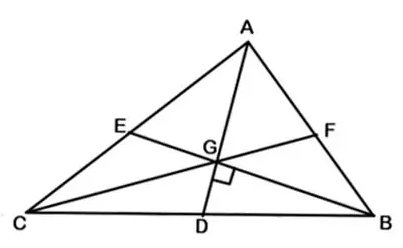### CAT 2019 – Slot 1 – Quantitative Ability – In a triangle ABC, medians AD and BE are perpendicular to each other

Q. 1:In a triangle ABC, medians AD and BE are perpendicular to each other, and have lengths 12 cm and 9 cm, respectively. Then, the area of triangle ABC, in sq cm, is
1. 80
2. 68
3. 72
4. 78Draw the third median CF. As We know that the :

1. The intersection point of medians i.e. centroid (G) divides each median into 2:1.

2. All three medians divide the triangle into 6 triangle having equal area.

So Area of Triangle ABC = 6* Area of one small triangle—————-1)

Now Given AD = 12 and GD:AG = 1:2 So GD = 1/3 *12 =4

BE = 9 and BG:GE = 2:1 So BG = 2/3 *9 = 6

As BG is perpendicular to GD,

Area of triangle BGD = ½ ×GB × GD = ½ × 6 × 4 =12

Hence, from eq 1) area of triangle ABC = 6 × 12 = 72

##### Q. 2: In 2010, a library contained a total of 11500 books in two categories – fiction and nonfiction. In 2015, the library contained a total of 12760 books in these two categories. During this period, there was 10% increase in the fiction category while there was 12% increase in the non-fiction category. How many fiction books were in the library in 2015?1. 60002. 61603. 55004. 6600

In 2010:

Fiction = x

Non fiction = 11500-x

In 2015:

Fiction = 1.1x

Non fiction = 1.2(11500-x)

But in 2015, total books are 12760

So, 1.1x + 1.2(11500-x) = 12760

On solving, we get Number of fiction books in the library in 2015 is 1.1x = 6600

Q. 3:The strength of a salt solution is p% if 100 ml of the solution contains p grams of salt. Each of three vessels A, B, C contains 500 ml of salt solution of strengths 10%, 22%, and 32%, respectively. Now, 100 ml of the solution in vessel A is transferred to vessel B. Then, 100 ml of the solution in vessel B is transferred to vessel C. Finally, 100 ml of the solution in vessel C is transferred to vessel A. The strength, in percentage, of the resulting solution in vessel A is
1. 12
2. 15
3. 14
4. 13

Volume of solution in each vessels = 500ml

Salt in each vessels , in A = 10% of 500 = 50 ml

In B = 22 % of 500 = 110 ml

In C = 32% of 500 = 160 ml

When 100 ml of the solution in vessel A is transferred to vessel B, salt in B = 110 + 10% of 100 = 110 + 10 = 120 ml

% tage of salt in B = 120/600 = 20%

So When 100 ml of the solution in vessel B is transferred to vessel C = 160 + 20% of 100 = 160 + 20 = 180

% tage of salt in C = 180/600 *100 = 30 %

Now when 100 ml of the solution in vessel C is transferred to vessel A , volume of solution in A = 400+100 = 500

Salt in A = 10% of 400 + 30% of 100 = 40 + 30 = 70

% tage of salt in A = 70/500 *100 = 14 %

Q. 4: How many factors of 2^4 x 3^5 x 10^4 are perfect squares which are greater than 1?

24 x 35 x 104 = 2^4×3^5×(2^4×5^4 )=2^8×3^5×5^4

Perfect square factors of the number will be of the form 2^a×3^b×5^c

Where a can be 0, 2, 4, 6 or 8 . b can be 0, 2 or 4 and c can be 0, 2 or 4

So total number of square factors = 5*3*3 = 45

But when a=b=c =0 factor will be 1.

Thus perfect squares which are greater than 1= 45 -1 = 44

Q. 5: What is the largest positive integer n such that n^2+7n+12 / n^2-n-12 is also a positive integer?
1. 6
2. 16
3. 8
4. 12

Given, (n^2+ 7n+12)/(n^2-n-12 )=(n^2+ 3n+4n+12)/(n^2-4n +3n-12)=((n+3)(n+4))/(n-4)(n+3) =(n+4)/(n-4) is an integers From the option we can see largest possible value of n =12

Inspiring Education… Assuring Success!!

Ghatkopar | Borivali | Andheri | Online